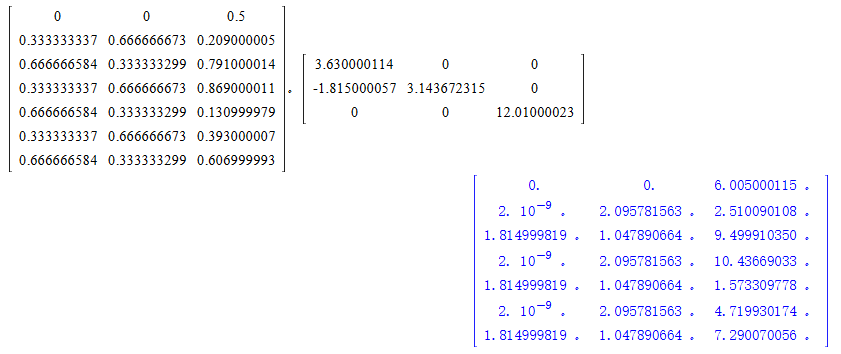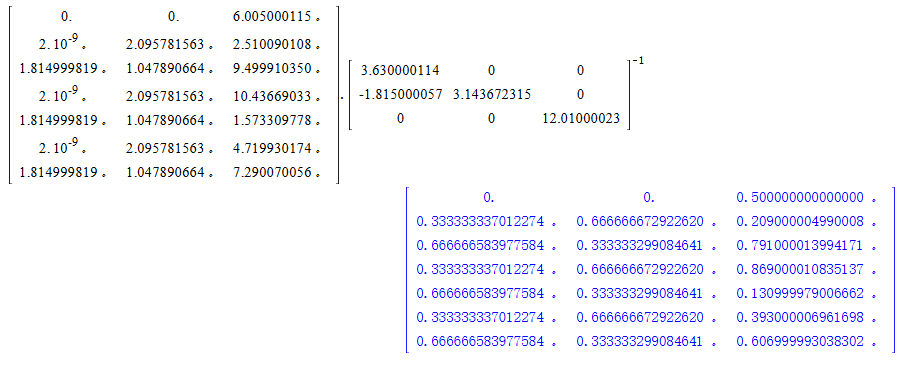# POSCAR中分数坐标与笛卡尔坐标相互转换

## 1. 分数坐标转笛卡尔坐标

$\begin{cases} x_i^{cart} = x_i^{frac} * x_A + y_i^{frac} * x_B + z_i^{frac} * x_C \\ y_i^{cart} = x_i^{frac} * y_A + y_i^{frac} * y_B + z_i^{frac} * y_C \\ z_i^{cart} = x_i^{frac} * z_A + y_i^{frac} * z_B + z_i^{frac} * z_C \end{cases}$

$\begin{cases} A(x_A, y_A, z_A) \\ B(x_B, y_B, z_B) \\ C(x_C, y_C, z_C) \end{cases}$## 2. 笛卡尔坐标转分数坐标